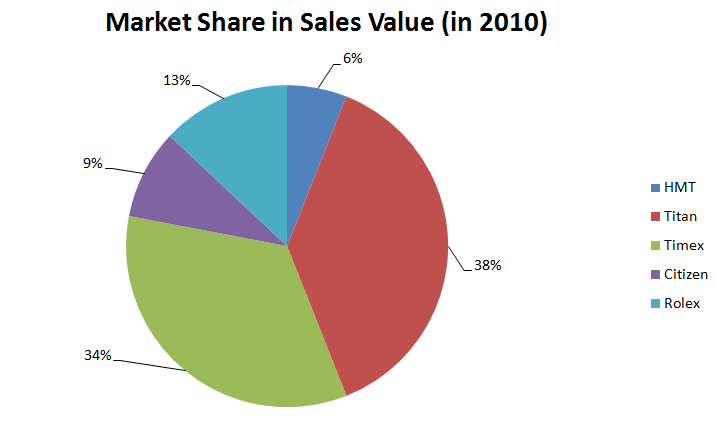New Students Offer - Use Code HELLO

# Data Interpretation Case Study - 3 Diagrams

Directions for Questions – Go through the data sets given below and solve the questions based on it.
Following data sets given below present the statistics related to the Indian watch industry.
There are five companies and their respective market share of the year 2010 is given in the pie chart given below.
Chart 1Following line chart presents the Titan Sales volume (in million units) for the years 2008 to 2012. All the values are even numbers.
Chart 2Following bar chart presents the average selling price (in Rs.) of these companies in the year 2010:

Chart 3(Assume there is no export or import)

Q1. For how many years, is it possible to calculate the size of domestic watch market (in Rs.)?
a) 0
b) 1
c) 2
d) 5
e) None of The Above

Q2. Which company has the second lowest sales (in Rs. terms) in the year 2010? a) Citizen
b) Rolex
c) HMT
d) Timex
e) None of The Above

Q3. Which year saw the lowest growth rate in number of unit sold over the previous year for Titan? a) 2009
b) 2010
c) 2011
d) 2012
e) None of The Above

Q4. What is the size of the domestic market in the year 2010 (in Rs.)?
a) Rs. 757 crores
b) Rs. 7578 crores
c) Rs. 75789 crores
d) Can’t be Determined
e) None of The Above

### Solution

Sales Value = Sales Volume × Average Price per Unit

Total Market Size :-

= Sales Value of Company X /Market Share (in %) of company X × 100

Question is asking for size of domestic market (in Rs.). Out of three different data sets given in the question (a pie chart, a line chart and a bar chart), we have Rupees value in only one data set – Bar chart. In pie chart and line chart, we do not have any information in Rupees Terms. So to answer any question in Rupees terms, we need to use Chart 3 – Bar Chart.

How do we calculate the size of domestic watch market:

Chart 1 provides the market share in sales volume in 2010. Chart 2 provides the sales volume of Titan from 2008 to 2012.

Using chart 2, Sales volume of Titan in 2010 = 32 million units and using chart 3, average selling price = Rs. 900/watch. So, total market value for Titan in 2010 = 32 million units × Rs. 900/watch = Rs. X (Assume) [we are not required to calculate this value as question is only asking for the possibility of market size calculation].

Now using chart I, market share of Titan is known = 38% = Rs. X. Using this, we can calculate 100% = Total market size of domestic watch market.

Hence it is possible to calculate only for the year 2010. We do not have Average Selling Price of Titan for the year other than 2010. So we can’t calculate the domestic market size for any year other than 2010.

Hence option B is the correct answer.

Now this is no brainer. You just have to calculate the percentage growth in the number of units sold of Titan.
2012 saw the minimum growth rate = 4.6%. Hence, option D is the answer.

Using the data from Answer 1,
So, total market value of Titan in 2010 = 32 million units × Rs. 900/watch = Rs. 28800 million
Titan has a market share of 38% in 2010.
Hence 38% = Rs. 28800 million
100% = Rs. 75789.47 million = Rs. 7578.9 crores
Hence, option B is the answer.